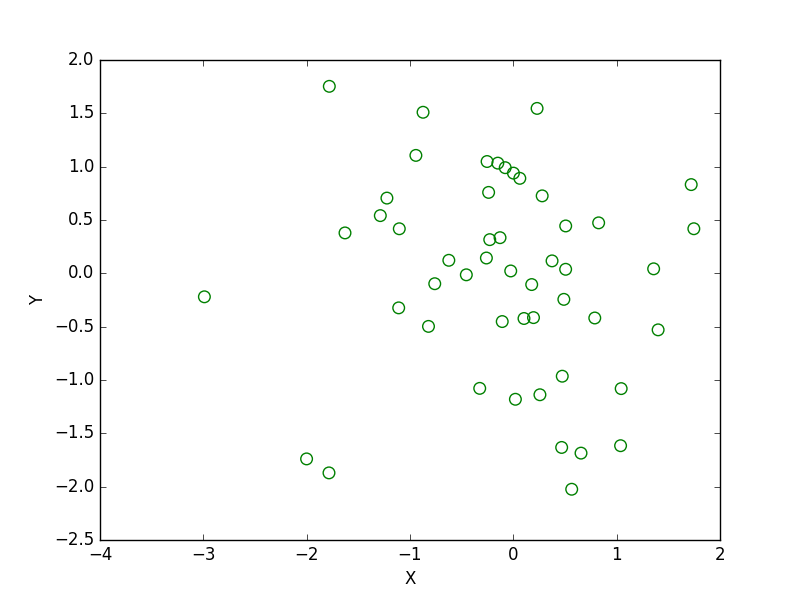﻿ Matplotlib Scatter: Draw a scatter plot with empty circles taking a random distribution in X and Y - w3resource# Matplotlib Scatter: Draw a scatter plot with empty circles taking a random distribution in X and Y

## Matplotlib Scatter: Exercise-2 with Solution

Write a Python program to draw a scatter plot with empty circles taking a random distribution in X and Y and plotted against each other.

Sample Solution:

Python Code:

``````import matplotlib.pyplot as plt
import numpy as np
x = np.random.randn(50)
y = np.random.randn(50)
plt.scatter(x, y, s=70, facecolors='none', edgecolors='g')
plt.xlabel("X")
plt.ylabel("Y")
plt.show()
```
```

Sample Output:Python Code Editor: